Скачать презентацию Modelling techniques and applications Qing Tan EPFL-STI-IMT-OPTLab 24

3462765efce89d3c5a224ee9cb0d3b0b.ppt

• Количество слайдов: 20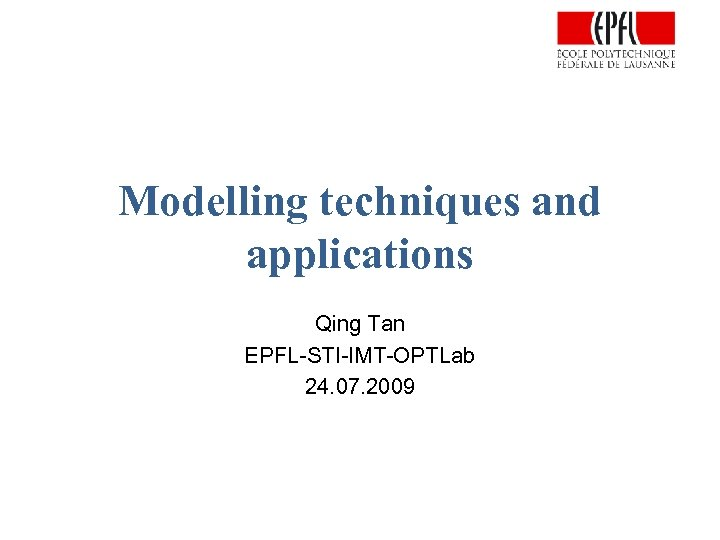Modelling techniques and applications Qing Tan EPFL-STI-IMT-OPTLab 24. 07. 2009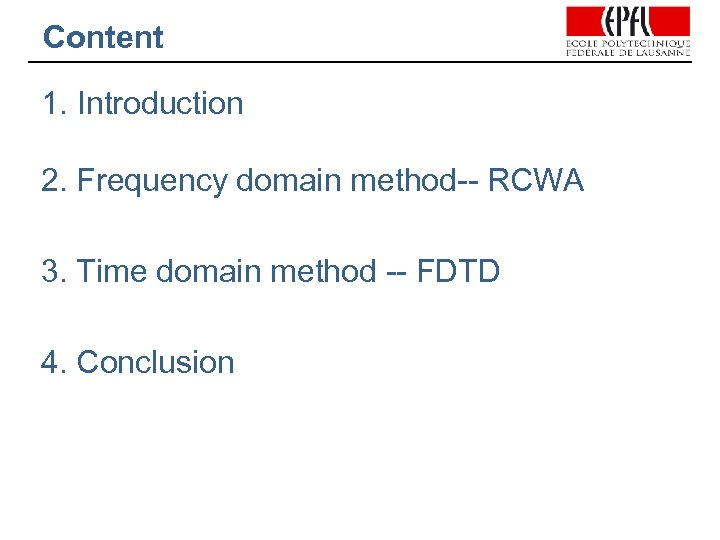Content 1. Introduction 2. Frequency domain method-- RCWA 3. Time domain method -- FDTD 4. Conclusion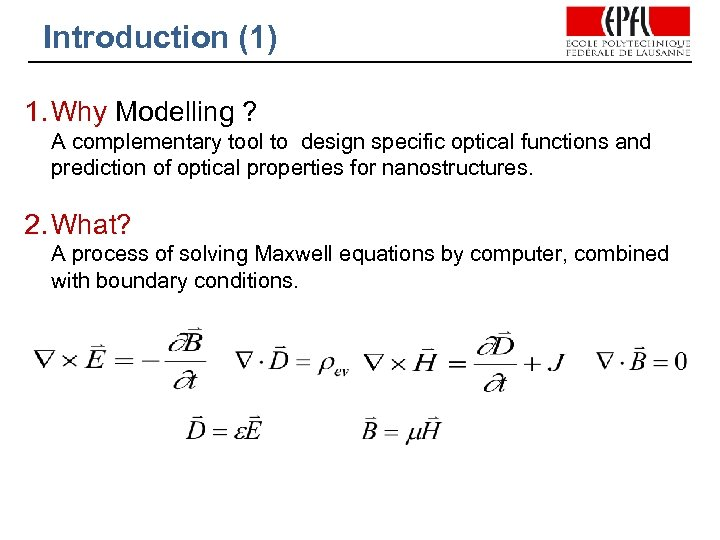Introduction (1) 1. Why Modelling ? A complementary tool to design specific optical functions and prediction of optical properties for nanostructures. 2. What? A process of solving Maxwell equations by computer, combined with boundary conditions.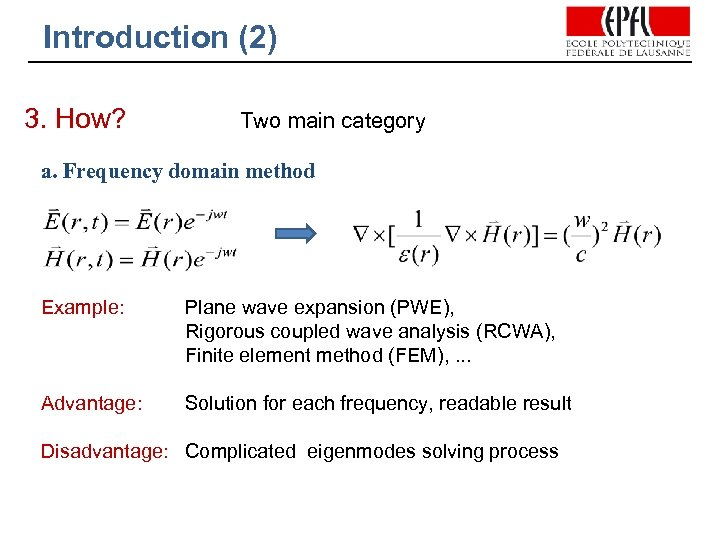Introduction (2) 3. How? Two main category a. Frequency domain method Example: Plane wave expansion (PWE), Rigorous coupled wave analysis (RCWA), Finite element method (FEM), . . . Advantage: Solution for each frequency, readable result Disadvantage: Complicated eigenmodes solving process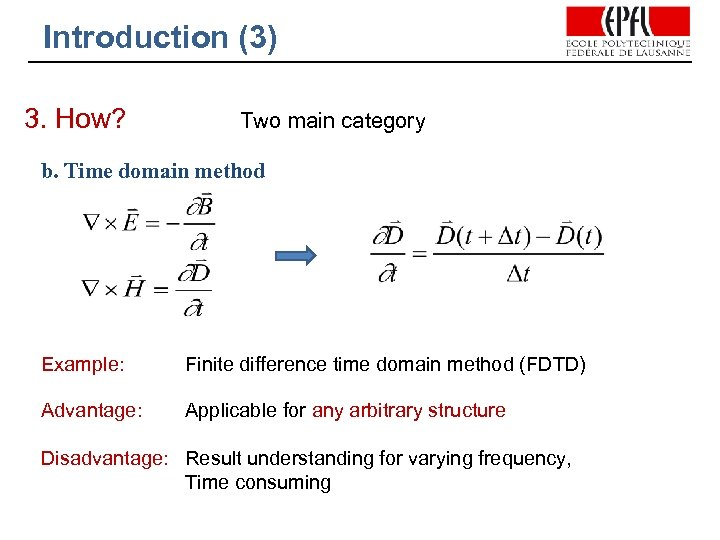Introduction (3) 3. How? Two main category b. Time domain method Example: Finite difference time domain method (FDTD) Advantage: Applicable for any arbitrary structure Disadvantage: Result understanding for varying frequency, Time consuming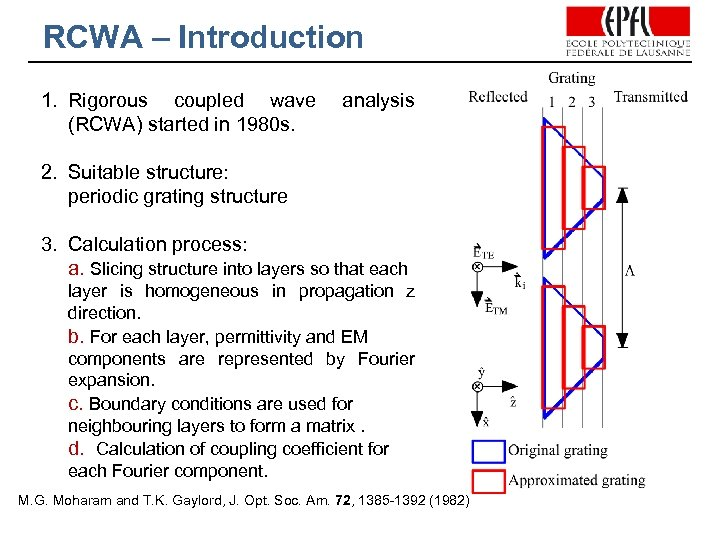RCWA – Introduction 1. Rigorous coupled wave (RCWA) started in 1980 s. analysis 2. Suitable structure: periodic grating structure 3. Calculation process: a. Slicing structure into layers so that each layer is homogeneous in propagation z direction. b. For each layer, permittivity and EM components are represented by Fourier expansion. c. Boundary conditions are used for neighbouring layers to form a matrix. d. Calculation of coupling coefficient for each Fourier component. M. G. Moharam and T. K. Gaylord, J. Opt. Soc. Am. 72, 1385 -1392 (1982)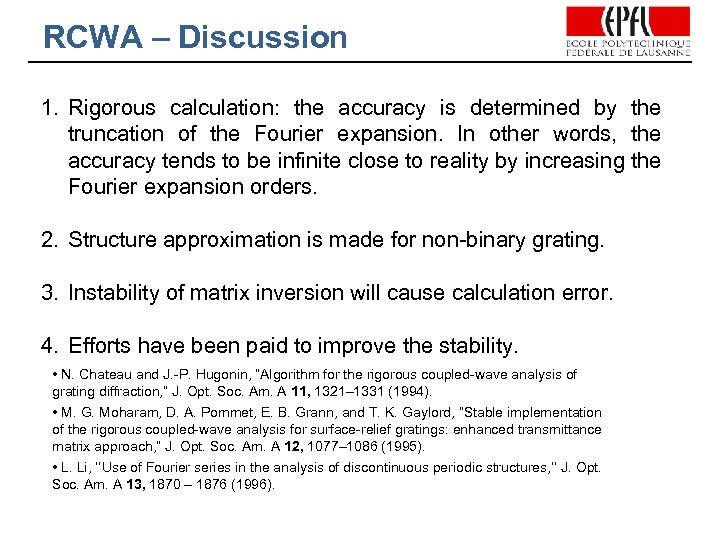RCWA – Discussion 1. Rigorous calculation: the accuracy is determined by the truncation of the Fourier expansion. In other words, the accuracy tends to be infinite close to reality by increasing the Fourier expansion orders. 2. Structure approximation is made for non-binary grating. 3. Instability of matrix inversion will cause calculation error. 4. Efforts have been paid to improve the stability. • N. Chateau and J. -P. Hugonin, “Algorithm for the rigorous coupled-wave analysis of grating diffraction, ” J. Opt. Soc. Am. A 11, 1321– 1331 (1994). • M. G. Moharam, D. A. Pommet, E. B. Grann, and T. K. Gaylord, “Stable implementation of the rigorous coupled-wave analysis for surface-relief gratings: enhanced transmittance matrix approach, ” J. Opt. Soc. Am. A 12, 1077– 1086 (1995). • L. Li, ‘‘Use of Fourier series in the analysis of discontinuous periodic structures, ’’ J. Opt. Soc. Am. A 13, 1870 – 1876 (1996).RCWA – Example (1) A polarizing beam splitter that uses the anisotropic spectral reflectivity (ASR) characteristics of a high spatial frequency multilayer binary grating. R. C. Tyan, P. C. Sun, Y. Fainman - SPIE MILESTONE SERIES MS, 2001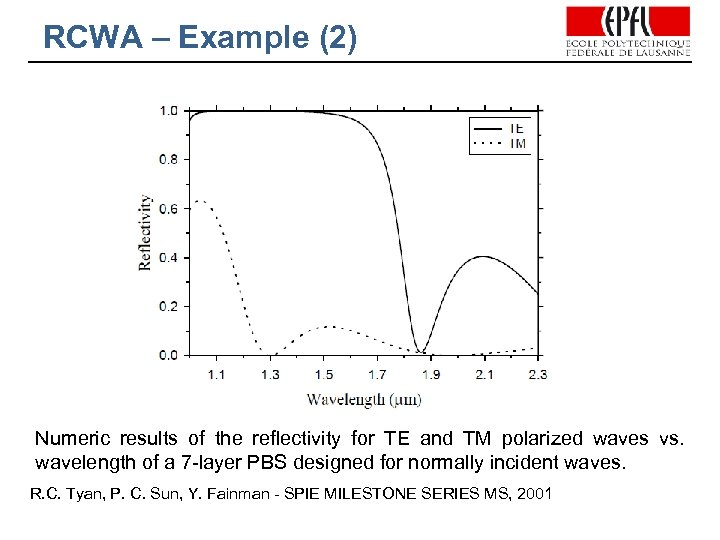RCWA – Example (2) Numeric results of the reflectivity for TE and TM polarized waves vs. wavelength of a 7 -layer PBS designed for normally incident waves. R. C. Tyan, P. C. Sun, Y. Fainman - SPIE MILESTONE SERIES MS, 2001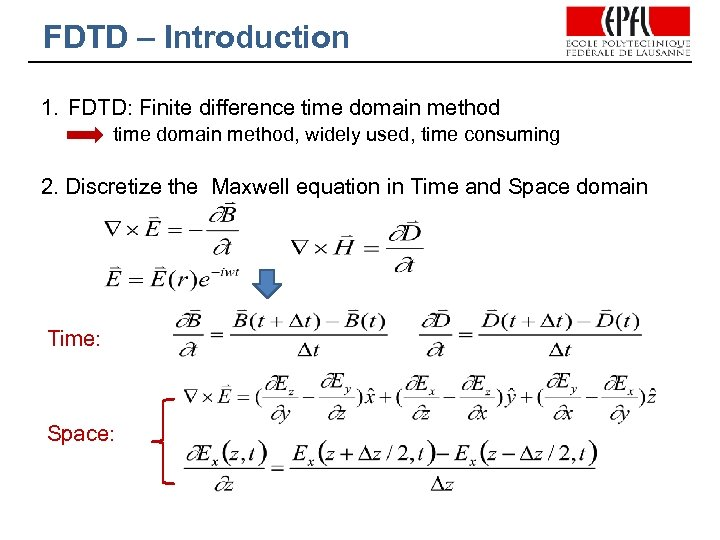FDTD – Introduction 1. FDTD: Finite difference time domain method, widely used, time consuming 2. Discretize the Maxwell equation in Time and Space domain Time: Space: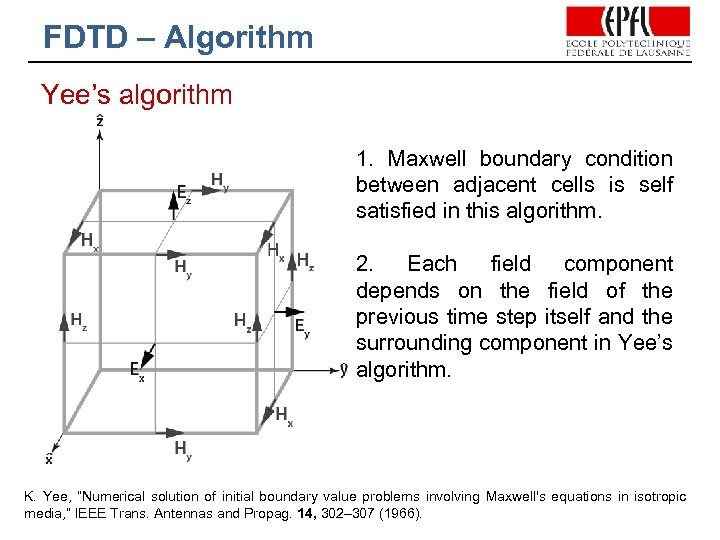FDTD – Algorithm Yee’s algorithm 1. Maxwell boundary condition between adjacent cells is self satisfied in this algorithm. 2. Each field component depends on the field of the previous time step itself and the surrounding component in Yee’s algorithm. K. Yee, “Numerical solution of initial boundary value problems involving Maxwell's equations in isotropic media, ” IEEE Trans. Antennas and Propag. 14, 302– 307 (1966).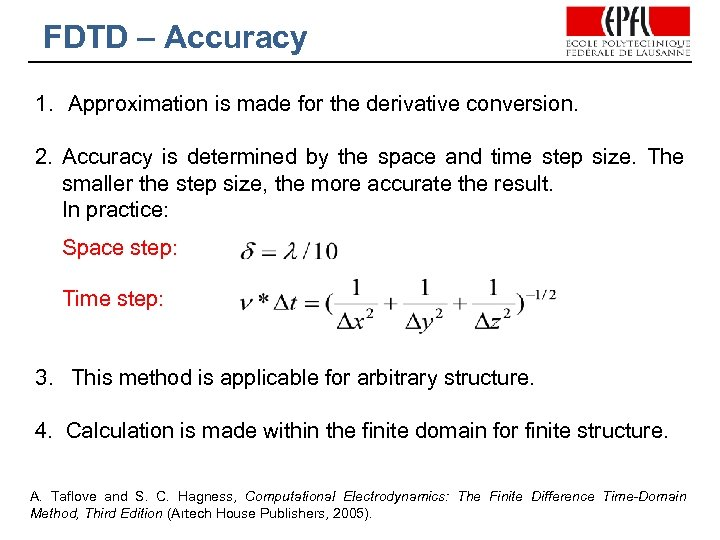FDTD – Accuracy 1. Approximation is made for the derivative conversion. 2. Accuracy is determined by the space and time step size. The smaller the step size, the more accurate the result. In practice: Space step: Time step: 3. This method is applicable for arbitrary structure. 4. Calculation is made within the finite domain for finite structure. A. Taflove and S. C. Hagness, Computational Electrodynamics: The Finite Difference Time-Domain Method, Third Edition (Artech House Publishers, 2005).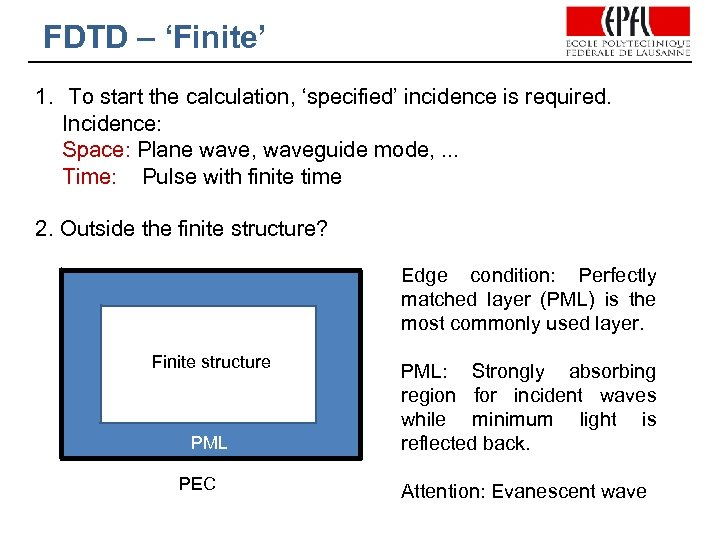FDTD – ‘Finite’ 1. To start the calculation, ‘specified’ incidence is required. Incidence: Space: Plane wave, waveguide mode, . . . Time: Pulse with finite time 2. Outside the finite structure? Edge condition: Perfectly matched layer (PML) is the most commonly used layer. Finite structure PML PEC PML: Strongly absorbing region for incident waves while minimum light is reflected back. Attention: Evanescent wave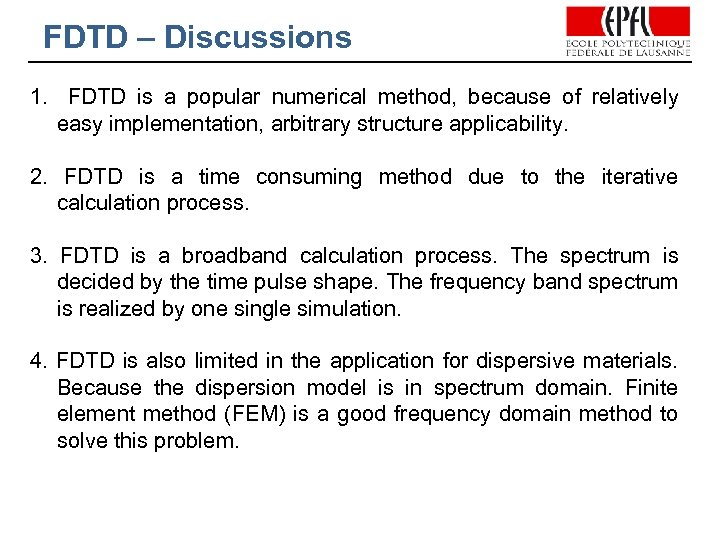FDTD – Discussions 1. FDTD is a popular numerical method, because of relatively easy implementation, arbitrary structure applicability. 2. FDTD is a time consuming method due to the iterative calculation process. 3. FDTD is a broadband calculation process. The spectrum is decided by the time pulse shape. The frequency band spectrum is realized by one single simulation. 4. FDTD is also limited in the application for dispersive materials. Because the dispersion model is in spectrum domain. Finite element method (FEM) is a good frequency domain method to solve this problem.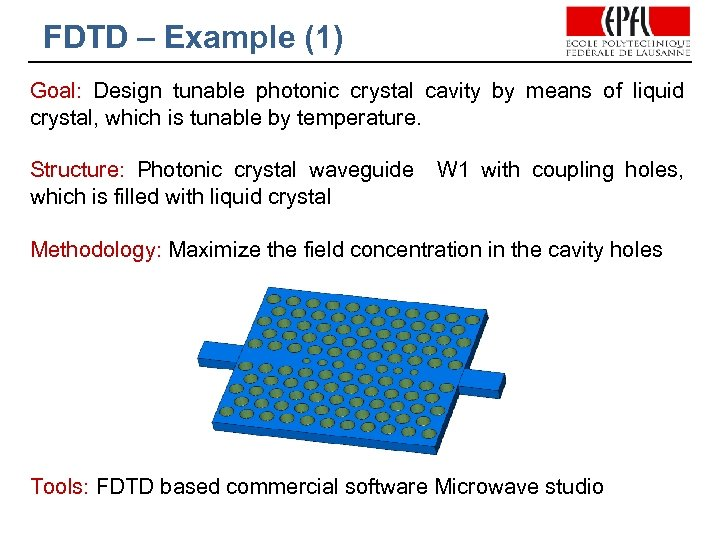FDTD – Example (1) Goal: Design tunable photonic crystal cavity by means of liquid crystal, which is tunable by temperature. Structure: Photonic crystal waveguide which is filled with liquid crystal W 1 with coupling holes, Methodology: Maximize the field concentration in the cavity holes Tools: FDTD based commercial software Microwave studio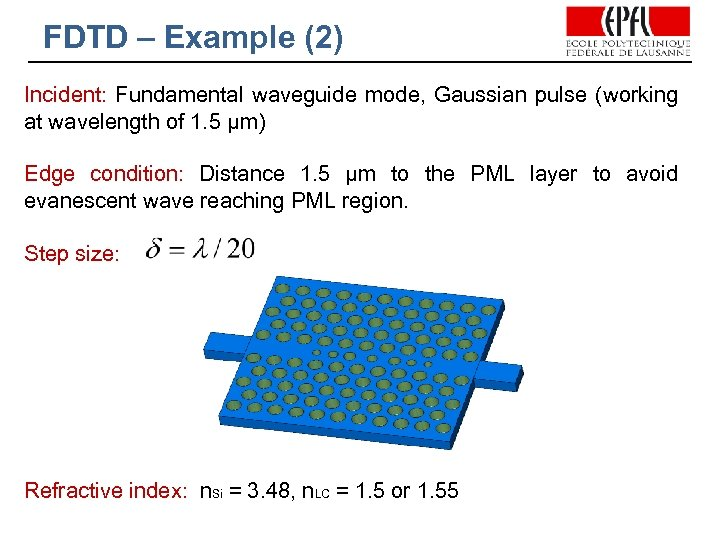FDTD – Example (2) Incident: Fundamental waveguide mode, Gaussian pulse (working at wavelength of 1. 5 µm) Edge condition: Distance 1. 5 µm to the PML layer to avoid evanescent wave reaching PML region. Step size: Refractive index: n. Si = 3. 48, n. LC = 1. 5 or 1. 55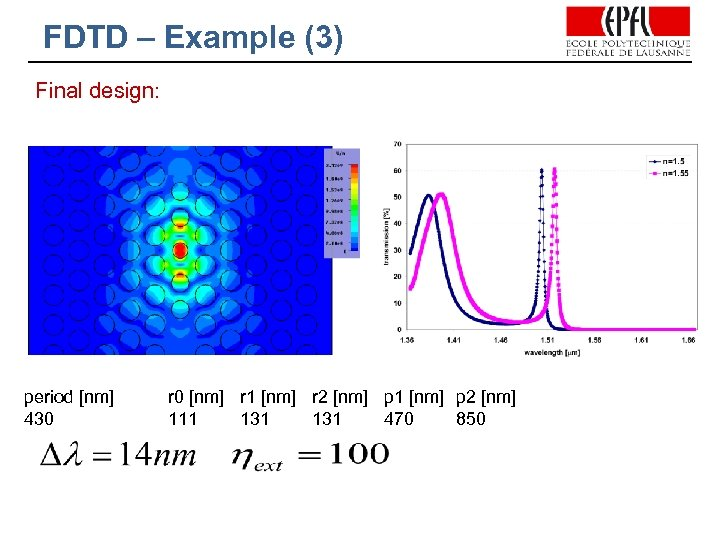FDTD – Example (3) Final design: period [nm] 430 r 0 [nm] r 1 [nm] r 2 [nm] p 1 [nm] p 2 [nm] 111 131 470 850FDTD – Example (4) Extinction ratio improvement: period [nm] 430 r 0 [nm] r 1 [nm] r 2 [nm] r 3 [nm] p 1 [nm] p 2 [nm] p 3[nm] 111 151 126 101 485 865 1295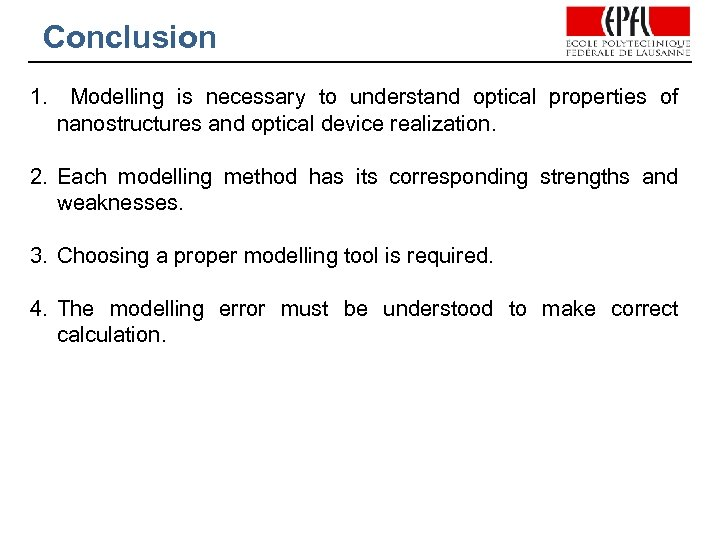Conclusion 1. Modelling is necessary to understand optical properties of nanostructures and optical device realization. 2. Each modelling method has its corresponding strengths and weaknesses. 3. Choosing a proper modelling tool is required. 4. The modelling error must be understood to make correct calculation.Thank you for your attention!